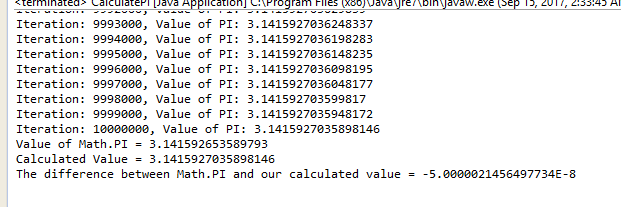# Question & Answer: This assignment will focus on the use of for( ) loops and while( ) loop…..

Java Assignment 4

 This assignment will focus on the use of for( ) loops and while( ) loops. Assignment 4
 Assignment 4 Submission Follow the directions below to submit Assignment 4: Don't use plagiarized sources. Get Your Custom Essay on Question & Answer: This assignment will focus on the use of for( ) loops and while( ) loop….. GET AN ESSAY WRITTEN FOR YOU FROM AS LOW AS \$13/PAGE Create a Java program. The class name for the program should be ‘CalculatePI’. In the main method you should perform the following: Create a for loop that will loop for 10,000,000 iterations that will be used to calculate the Mathematical value of PI. You will need a variable to count the iterations. You will need a variable that will be used in the mathematical calculation inside the loop. The formula for PI is: PI = 4 * (1 – 1/(2*i-1) + 1/(2*i+1) . . . Each 1000th iteration of the loop should print the iteration number and the currently calculation for PI. At the end of the loop the program should display the value of PI as determined by the variable Math.PI and the difference between your calculated value and the value contained in Math.PI You should then repeat the instructions above using a while( ) loop rather than a for( ) loop. Pseudo flowchart for the assignment program: Create a variable to contain the PI calculation. The initial value of this variable should be 1. Create a for( ) loop that will loop for 10,000,000 iterations. You will need a loop variable to count the number iterations. Create a second loop variable that will be used for i in the calculation. The initial value of this varaible should be 2. For each iteration of the loop you will need to subtract the value of 1.0/(2*i-1) from the calculation and add the value of 1.0/(2*i+1). At the end of the loop you will increment the iteration loop variable by 1 and increment the value of i used in the calculation by 2. Every 1000th iteration of the loop you should display the iteration number and the calculated value for PI. When you have completed the loop you should print the value of Math.PI and the difference between Math.PI and your calculated value of PI. You will then repeat this process using a while( ) loop.With For loop:

public class CalculatePI {

public static void main(String args[]){

double PI=1d;

for(int k=0,i=2;k<10000000;k++,i=i+2){

PI-=1.0/((2*i)-1);

PI+=1.0/((2*i)+1);

if((k+1)%1000 == 0){

System.out.println(“Iteration: ” + (k+1) + “, Value of PI: “+ (4*PI));

}

}

System.out.println(“Value of Math.PI = “+Math.PI );

System.out.println(“Calculated Value = ” + (4 * PI));

System.out.println(“The difference between Math.PI and our calculated value = ” + (Math.PI – (4 * PI)));

}

}

Sample Output: (Only last few lines)

.

.

.

.

.

Iteration: 9998000, Value of PI: 3.141592703599817

Iteration: 9999000, Value of PI: 3.1415927035948172

Iteration: 10000000, Value of PI: 3.1415927035898146

Value of Math.PI = 3.141592653589793

Calculated Value = 3.1415927035898146

The difference between Math.PI and our calculated value = -5.0000021456497734E-8

With while loop:

public class CalculatePI {

public static void main(String args[]){

double PI=1;

int i=2;

int k=0;

while(k<10000000){

PI-=1.0/((2*i)-1);

PI+=1.0/((2*i)+1);

if((k+1)%1000 == 0){

System.out.println(“Iteration: ” + (k+1) + “, Value of PI: “+ (4*PI));

}

k++;

i=i+2;

}

System.out.println(“Value of Math.PI = “+Math.PI );

System.out.println(“Calculated Value = ” + (4 * PI));

System.out.println(“The difference between Math.PI and our calculated value = ” + (Math.PI – (4 * PI)));

}

}

Sample output: (Only last few lines)

.

.

.

.

.

Iteration: 9998000, Value of PI: 3.141592703599817

Iteration: 9999000, Value of PI: 3.1415927035948172

Iteration: 10000000, Value of PI: 3.1415927035898146

Value of Math.PI = 3.141592653589793

Calculated Value = 3.1415927035898146

The difference between Math.PI and our calculated value = -5.0000021456497734E-8

Kindly let me know if you have any doubts.